Courses

# Computer Science And Information Technology - CS 2017 GATE Paper - 1 (Practice Test)

## 65 Questions MCQ Test GATE Past Year Papers for Practice (All Branches) | Computer Science And Information Technology - CS 2017 GATE Paper - 1 (Practice Test)

Description
This mock test of Computer Science And Information Technology - CS 2017 GATE Paper - 1 (Practice Test) for GATE helps you for every GATE entrance exam. This contains 65 Multiple Choice Questions for GATE Computer Science And Information Technology - CS 2017 GATE Paper - 1 (Practice Test) (mcq) to study with solutions a complete question bank. The solved questions answers in this Computer Science And Information Technology - CS 2017 GATE Paper - 1 (Practice Test) quiz give you a good mix of easy questions and tough questions. GATE students definitely take this Computer Science And Information Technology - CS 2017 GATE Paper - 1 (Practice Test) exercise for a better result in the exam. You can find other Computer Science And Information Technology - CS 2017 GATE Paper - 1 (Practice Test) extra questions, long questions & short questions for GATE on EduRev as well by searching above.
QUESTION: 1

### Find the smallest number y such that y x 162 (y multiplied by 162) is a perfect cube.

Solution:

Explanation:

162 = 3 * 3 * 3 * 3 * 2 To make it a perfect cube, we need at least 2 * 2 * 3 * 3 which is 36.

Alternate Solution

Take the prime factor of 162: 162 = 2 * 81 = 2 * 9 * 9 = 2 * 3 * 3 * 3 * 3, now if we add minimum two 2 and two 3, then it will be perfect cube of a number. Therefore, the value of y will be = 2 * 2 * 3 * 3 = 4 * 9 = 36 Note that you can multiple 162 with values in given options, then take cube root with the help of calculator for each option, the answer should be an integer.

QUESTION: 2

### Rahul, Murali, Srinivas and Arul are seated around a square table. Rahul is sitting to the left of Murali. Srinivas is sitting to the right of Arul. Which of the following pairs are seated opposite each other ?

Solution:

Assume, they are looking to the center of circular table, then the possible arrangement is: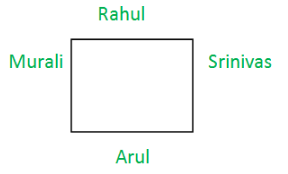QUESTION: 3

### Research in the workplace reveals thatpeople work for many reasons _________.

Solution:

Grammatically, besides is an adverb or a preposition, and beside a preposition. Beside means next to. As a preposition, besides means in addition to or apart from. As an adverb, besides means as well or furthermore.
Here, we use "besides" as a preposition meaning "in addition to".
"besides money" is correct choice.

QUESTION: 4

The probability that a k-digit number does NOT contain the digits 0, 5 or 9 is

Solution:

For 10 digits(0 to 9) total possible cases = (10)k
Excluding digits 0, 5, 9, total possible cases = (7)k with numbers 1,2,3,4,6,7,8
Required probability = (7)k/(10)k= (0.7)
Therefore option C is correct

Alternate Solution
Case(1): When 0 can not be as left most digit:
Then sample space = 9 * 10^{k-1}
Favorable event = 7^{k}
Required probability = (7^{k}) / (9 * 10^{k-1}).
None option matches.
Case(2): When 0 can be as left most digit:
Then sample space = 10^{k}
Favorable event = 7^{k}
Required probability = (7^{k}) / (10^{k}) = (7/10)^{k} = (0.7)^{k}.
Option (c) matches.

QUESTION: 5

After Rajendra Chola returned from his voyage to Indonesia, he ______ to visit the temple in Tanjavur.

Solution:

According to the rule : when the main clause is in the past or past perfect tense, the subordinate clause must be in the past or past perfect tense.

QUESTION: 6

Six people are seated around a circular table. There are at least two men and two women. There are at least three right-handed persons. Every woman has a left-handed person to her immediate right. None of the women are right-handed. The number of women at the table is

Solution:

Let's draw what these statements say:

• There are atleast Two Men and Two Women.
• There are atleast 3 Right Handed People
• No women are Right Handed - infers there are three right handed men
• Every woman has a left-handed person to her immediate right - Means women circled in red needs to have a left handed person on her right and that too a man as on right side of ?? there is a right handed man.
• So,  ?? - will be a left handed man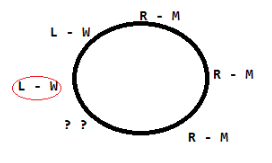Therefore, option A (only 2 women) is correct

QUESTION: 7

"The hold of the nationalist imagination on our colonial past is such that anything inadequately or improperly natinalist is just not history"

Q. Which of the following statements best reflects the author's opinion?

Solution:

The author is trying to say that everything related to colonial past has a hold of nationalism in people’s imagination. It is not necessary that anything which is represented inadequately is the history. History and nationalism has a strong connection, but sometimes history is viewed with nationalism in mind.

QUESTION: 8

The expression [(x + y) - |x - y|] / 2 is equal to

Solution:

As we know that, if x > y, then |x - y| = x - y and
if x < y then |x - y| = y - x , because value of |x - y| is always non-negative.
Therefore,

• Case 1: If x > y : (x + y) - |x - y|] / 2 =  (x + y) - (x - y)] / 2 = 2y / 2 = y (Minimum of x , y)
• Case 2: If x < y : (x + y) - |x - y|] / 2 = (x + y) - (y - x)] / 2 = 2x / 2 = x (Minimum of x , y)

Therefore in both the case we get minimum of (x,y). So, option B
Note that you can take some random values of x and y, then verify given options.

QUESTION: 9

A contour line joins locations having the same height above the mean sea level. The following is a contour plot of a geographical region. Contour lines are shown at 25 m intervals in this plot.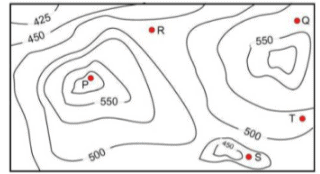Q. If in a flood, the water level rises to 525 m, which of the villages P, Q, R, S, T get submerged?

Solution:

Given data - height of all villages

• P = 575
• Q = 525
• R = 475
• S = 425
• T = 500

Villages  below the flood level - 525m are

1. R <500m
2. 425m<= S<=450m
3. 500m <=T<= 525m

Therefore option C is correct.

QUESTION: 10

Arun, Gulab, Neel and Shweta must choose one shirt each from a pile of four shirts coloured red, pink, blue and white respectively. Arun dislikes the colour red and Shweta dislikes the colour white. Gulab and Neel like all the colours. In how many different ways can they choose the shirts so that no one has a shirt with a colour he or she dislikes?

Solution:

Total number of Shirts = 4 , Total candidates =4

• So total combination of possibilities = 4 X 4 = 16
• No.of ways  'Arun chooses red' + No.of ways Shwetha chooses white '=  2

Therefore, Answer is 16-2 = 14

QUESTION: 11

Let X be a Gaussian random variable with mean 0 and variance σ2. Let Y = max(X,0) where max(a,b) is the maximum of a and b. The median of Y is _____.

Note: This questions appeared as Numerical Answer Type.

Solution:

Here, half of the values of Y are to the left of the mean X = 0 and the remaining half of the values of Y lies to the right of the mean X = 0. hence,The median of Y = 0.

QUESTION: 12

Consider a TCP client and a TCP server running on two different machines. After completing data transfer, the TCP client calls close to terminate the connection and a FIN segment is sent to the TCP server. Server-side TCP responds by sending an ACK which is received by the client-side TCP. As per the TCP connection state diagram(RFC 793), in which state does the client side TCP connection wait for the FIN from the server-side TCP?

Solution:

The given scenario can be illustrated as: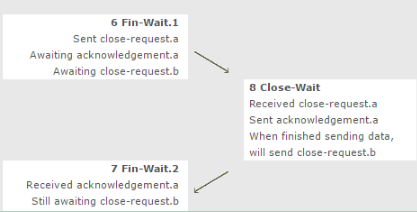QUESTION: 13

A sender S sends a message m to receiver R, which is digitally signed by S with its private key. In this scenario, one or more of the following security violations can take place.

(I) S can launch a birthday attack to replace m with a fraudulent message.

(II) A third party attacker can launch a birthday attack to replace m with a fraudulent message.

(III) R can launch a birthday attack to replace m with a fraudulent message.

Solution:
QUESTION: 14

Consider the following intermediate program in three address code

p = a - b
q = p * c
p = u * v
q = p + q

Q. Which one of the following corresponds to a static single assignment from the above code?

Solution:

According to Static Single Assignment

1. A variable cannot be used more than once in the LHS
2. A variable should be initialized atmost once.

Now looking at the given options

• a - code violates condition 1 as p1 is initialized again in this statement: p1 = u * v
• c- code is not valid as  q1 = p2 * c , q2 = p4 + q3 - In these statements p2, p4, q3 are not initialized anywhere
• d- code is invalid as  q2 = p + q is incorrect without moving it to register

Therefore, option B is only correct option.

QUESTION: 15

The following functional dependencies hold true for the relational schema R{V, W, X, Y, Z}:

V -> W
VW -> X
Y -> VX
Y -> Z

Q. Which of the following is irreducible equivalent for this set of functional dependencies?

Solution:

Given

V -> W
VW -> X
Y -> VX
Y -> Z

We need to find the minimal cover of these FDs
Option B. W->X can  not implied by the given FDs, so incorrect
Option C. Y->X can be implied from Y->V and V->X, hence redundant
Option D. W->X can  not implied by the given FDs, so incorrect
Option A. Minimal cover of dependencies that can be extracted out as

1. V -> W
2. V -> X
3. Y -> V
4. Y -> Z

Therefore, option A is most appropriate.

Alternate Solution

Irreducible equivalent functional dependency is minimal cover. Given,

{V → W, VW → X, Y → VX, Y → Z}

W extraneous from VW, since we have V → W,

= {V → W, V → X, Y → VX, Y → Z}

X extraneous from VX, since we have V → X,

= {V → W, V → X, Y → V, Y → Z}

QUESTION: 16

Consider the following CPU processes with arrival times (in milliseconds) and length of CPU bursts (in milliseconds) as given below: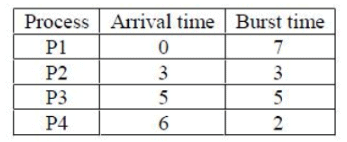Q. If the pre-emptive shortest remaining time first scheduling algorithm is used to schedule the processes, then the average waiting time across all processes is _______ milliseconds.

Solution: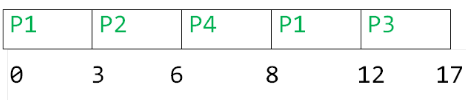Turn Around Time

P1  = 12-0 = 12
P2 = 6-3 = 3
P3 = 17-5 = 12
P4 = 8 - 6 = 2

Waiting Time

P1  = 12-7 = 5
P2 = 3-3 = 0
P3 = 12-5 = 7
P4 = 2 - 2 = 0

Average Waiting time = (7+0+5+0)/4 = 3.0 Therefore, option C is correct

Alternate Solution

Given, with arrival time and burst time: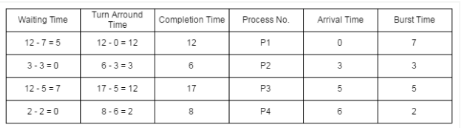Using (preemptive) shortest remaining time first algorithm, gantt chart is: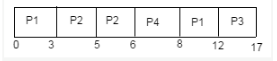Therefore, Average waiting time = (5 + 0 + 7 + 0)/4 = 12/4 = 3

QUESTION: 17

Let T be a binary search tree with 15 nodes. The minimum and maximum possible heights of T are:

Note: The height of a tree with a single node is 0.

Solution:

The minimum height of a binary search tree will be when tree is full complete tree: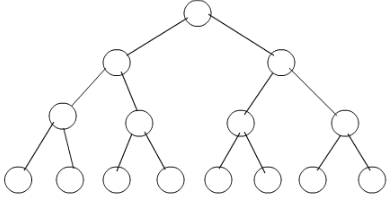Now, let h be the height of the binary tree, then, 2^{0}+2^{1}+2^{2}+2^{3}+...+2^{h}=2^{h+1}-1 <= n
So, Min height of a binary search tree = log2(n+1) - 1 = log2(15+1) - 1 = 4 - 1 = 3

The maximum height of a binary search tree will be when the tree is fully skewed: (like below)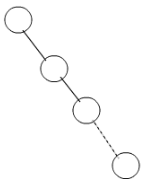Max height of the binary search tree = n-1 = 15 - 1 = 14, where the tree is Skewed tree

Therefore, option B

QUESTION: 18

The statement (¬ p) => (¬ q) is logically equivalent to which of the statements below?

I. p => q
II. q => p
III. (¬ q) ∨ p
IV. (¬ p) ∨ q

Solution:

(¬ p) => (¬ q) is logically equivalent  ¬ q + p
I. p => q ≡  ¬ p +  q
II. q => p ≡ ¬ q + p
III. (¬ q) ∨ p ≡ ¬ q + p
IV. (¬ p) ∨ q ≡ ¬ p + q
Therefore option D, II and III only

QUESTION: 19

Consider a database that has the relation schema EMP (EmpId, EmpName, and DeptName). An instance of the schema EMP and a SQL query on it are given below.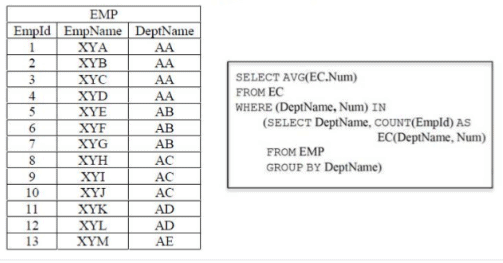Q. The output of executing the SQL query is _______.

Note: This questions appeared as Numerical Answer Type.

Solution:

Output of Query will be: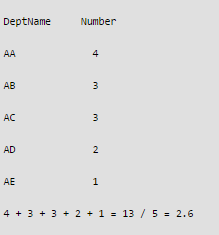QUESTION: 20

Threads of a process share

Solution:

Thread share all other  resources of process except local data like  - register , stack.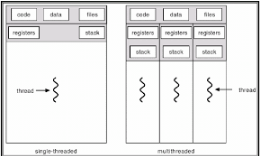QUESTION: 21

Let c1,cn be scalars not all zero. Such that the following expression holds: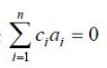where ai is column vectors in Rn. Consider the set of linear equations.
Ax = B.
where A = [a1.......an] and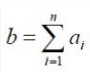Q. Then, Set of equations has

Solution:

The vectors a1, a2, …, an are linearly dependent.
For the system AX = B,
Rank of coefficient matrix A = Rank of augmented matrix (A / B)
= k (k< n)
Hence, the system has infinitely many solutions.

QUESTION: 22

Consider the first-order logic sentence

F: ∀ x (∃ y R(x,y)).

Q. Assuming non-empty logical domains, which of the sentences below are implied by F?

I. ∃y (∃x R(x,y))
II. ∃y (∀x R(x,y))
III. ∀y (∃x R(x,y))
IV. ∼∃x (∀y R(x,y))

Solution:

Given, first order logic sentence
F: ∀x (∃y R(x, y)) with following sentences:
(i) ∃y (∃x R(x, y)) is true. Because we have ∀x (∃y R(x, y)) → ∃x (∃y R(x, y)) → ∃y (∃x R(x, y)).
(ii) ∃y (∀x R(x, y)) is false. Because we have ∀x (∃y R(x, y)) ← ∃y (∀x R(x, y)).
(iii) ∀y (∃x R(x, y)) is false. Because for ∃y can not imply ∀y.
(iv) ∼∃x (∀y ∼R(x, y)) is true. Because ∼∃x (∀y ∼R(x, y)) = ∀x (∼ ∃y ∼R(x, y)) = ∀x (∃y ∼∼R(x, y)) = ∀x (∃y R(x, y)).

QUESTION: 23

Consider the following grammar

p --> xQRS
Q --> yz|z
R --> w|∈
S -> y

Q. Which is FOLLOW(Q)?

Solution: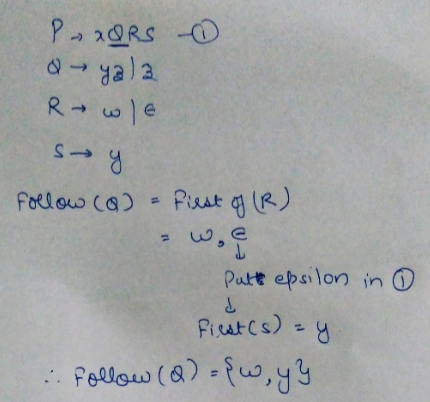Alternate Solution

To compute FOLLOW(A) for all non terminals A, apply the following rules until nothing can be added to any FOLLOW set:

• Place \$ in FOLLOW(S), where S is the start symbol and \$ is the input right end marker.
• If there is a production A → αBβ, then everything in FIRST(β), except for ∈, is placed in FOLLOW(B)
• If there is a production A → αB, or a production A → αBβ where FIRST(β) contains ∈ (i.e., b → ∈), then everything in FOLLOW(A) is in FOLLOW(B).

Therefore, follow(Q) = First(RS) = ( w ) ∪ First(S) = { w } ∪ { y } = { w,y }.
Note that here, first(S) is not ∈.

QUESTION: 24

Consider a two-level cache hierarchy L1 and L2 caches. An application incurs 1.4 memory accesses per instruction on average. For this application, the miss rate of L1 cache 0.1, the L2 cache experience on average. 7 misses per 1000 instructions. The miss rate of L2 expressed correct to two decimal places is ______________.

Note: This questions appeared as Numerical Answer Type.

Solution:

Given, Memory reference/instruction = 1.4
So, total number of memory references = 1000 * 1.4 = 1400
No. of  7 misses in L2 cache = 7/1400 = 0.005
Miss rate of L2=No. of misses in L2/No. of misses in L1 =0.005/0.1 =0.05
Therefore, option A is correct.

Alternate Solution

On Average, 1.4 memory accesses are required for one instruction execution.
So, for 1000 instructions, 1400 accesses are needed.
Number of misses occurred in cache L2 for 1000 instruction = 7/1400 = 0.005
Miss rate of L2 cache = misses occured in L2 cache / miss rate in L1 cache
= 0.005 / 0.1 = 0.05 (A)

QUESTION: 25

Consider the following functions from positives integers to real numbers 10, √n, n, log2n, 100/n. The CORRECT arrangement of the above functions in increasing order of asymptotic complexity is:

Solution:

For the large number, value of inverse of number is less than a constant and value of constant is less than value of square root.
Therefore, 100/n < 10< log<sub>2</sub>n< √n< n
It can be proven by substituting any big number in all the functions.

QUESTION: 26

Consider the C struct defines below: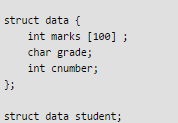Q. The base address of student is available in register R1. The field student.grade can be accessed efficiently using

Solution:

The address of student grade can only be accessed by using the base address of Student.

• Post-increment addressing mode. (R1)+ - Will give the next address and not the desired grade address
• Pre-decrement addressing mode, -(R1) - Will give the previous address and not the desired grade address
• Register direct addressing mode, R1 : In this mode, the address of the operand is embedded in the instruction code.
• Index addressing mode: It is the only mode which gives access to next address by adding displacement in base address using displacement mode. . Base register contains a pointer to a memory location. An integer (constant) is also referred to as a displacement. The address of the operand is obtained by adding the contents of the base register plus the constant.

Therefore, option D is correct

QUESTION: 27

Consider the following C code: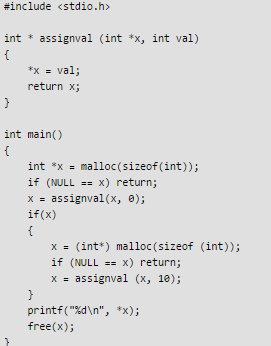Q. The code suffers from which one of the following problems:

Solution:

The code will run and give output = 10, so option A and B are discarded.

int * x= malloc (sizeof(int));

This statement assigns a location to x. Now,

(int*)malloc(sizeof(int));

again assigns a new location to x , previous memory location is lost because now we have no reference to that location resulting in memory leak. Therefore, option D is correct. Memory leak occurs when programmers create a memory in heap and forget to delete it. Memory leaks are particularly serious issues for programs like daemons and servers which by definition never terminate. Detailed article on Memory LeakRunning code of the question: [sourcecode language="C"] #include int *assignval (int *x, int val) { *x = val; return x; } void main () { int *x = malloc(sizeof(int)); if (NULL == x) return; x = assignval (x,0); if (x) { x = (int *)malloc(sizeof(int)); if (NULL == x) return; x = assignval (x,10); } printf("%d ", *x); free(x); } [/sourcecode]

QUESTION: 28

Consider the Karnaugh map given below, where X represents “ don’t care” and blank represents 0.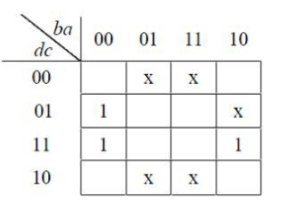Q. Assume for all inputs (a, c, d) the respective complements (a', b', c', d')are also available. The above logic is implemented 2-input NOR gates only. The minimum number of gates required is ____________.

Solution: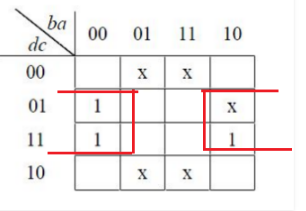Solving the above k- map, = ca'(b+b') = ca' This can be implemented using one NOR gate. Therefore, option A is correct

QUESTION: 29

Consider the following table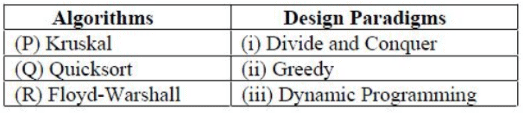Q. Match the algorithm to design paradigms they are based on:

Solution:
• Kruskal’s  is a greedy technique of Minimum Spanning Tree Algorithm to find an edge of the least possible weight that connects any two trees in the forest.

• QuickSort is a Divide and Conquer algorithm. It picks an element as pivot and partitions the given array around the picked pivot.
• Floyd Warshall Algorithm is for solving the All Pairs Shortest Path problem using Dynamic Programming. The problem is to find shortest distances between every pair of vertices in a given edge weighted directed Graph.
QUESTION: 30

Consider the C code fragment given below.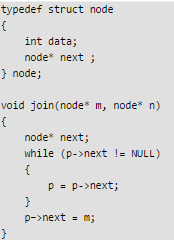Q. Assuming that m and n point to valid NULL- terminated linked lists, invocation of join will

Solution:

As it is stated in the question, that m and n are valid Lists but not explicitly specified if the lists are empty or not. We can have two cases:

1. Case 1: If lists are not NULL : Invocation of join will append list m to the end of list n if the lists are not NULL. For Example:Before join operation : m =1->2->3->4->5->null n =6->7->8->9->nullAfter join operation : 6->7->8->9->1->2->3->4->5->null
2. Case 2: If lists are NULL : If the list n is empty and itself NULL, then joining and referencing would obviously create NULL pointer issue.

Therefore option B is correct

QUESTION: 31

Consider the language L given by the regular expression (a + b) *b(a +b) over the alphabet {a, b}. The smallest number of states needed in a deterministic finite-state automaton (DFA) accepting L is ______.

Note: This questions appeared as Numerical Answer Type.

Solution: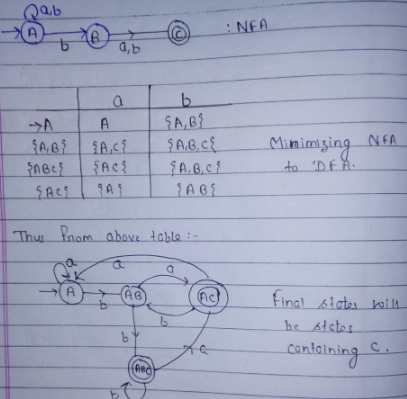QUESTION: 32

The n-bit fixed-point representation of an unsigned real number X uses f bits for the fraction part. Let i = n - f. The range of decimal values for X in this representation is

Solution:

Since given number is in unsigned bit representation, its decimal value starts with 0.
We have i bit in integral part so maximum value will be 2i
Thus integral value will be from 0 to 2i - 1
We know fraction part of binary representation are calculated as (1/0)*2-f
Thus with f bit maximum number possible = sum of GP series with a = 1/2 and r = 1/2

Thus fmax = {1/2(1 – (1/2)f}/(1 – 1/2) = 1 – 2-f

Thus maximum fractional value possible = 2i – 1 + (1 – 2-f)

= 2i - 2-f

QUESTION: 33

Let T be a tree with 10 vertices. The sum of the degrees of all the vertices in T is _____.

Note: This questions appeared as Numerical Answer Type.

Solution:

Given, v= Total vertices = 10 e = v - 1 =9 Degree = 2 * e = 18 Therefore, option A is correct

QUESTION: 34

Consider the following context-free grammar over the alphabet ∑ = {a, b, c} with S as the start symbol:

S -> abScT | abcT

T -> bT | b

Q. Which of the following represents the language generated by the above grammar?

Solution:
QUESTION: 35

When two 8-bit numbers A7 ... A0 and B7 ... B0 in 2's complement representation (with A0 and B0 as the least significant bits) are added using ripple-carry adder. the sum bits obtained are S7 ... S0and the carry bits are C7 ... C0. An overflow is said to have occured if

Solution:

Overflow indicates that the result was too large or too small to fit in the original data type.
Overflow flag indicates an overflow condition for a signed operation. Signed numbers are represented in two's complement representation.
The overflow occurs only when two positive number are added and the result is negative or two negative number are added and the result is positive. Otherwise, the sum has not overflowed.
Therefore, a XOR operation can quickly determine if an overflow condition exists. i.e.,
(A7 . B7)⊕(S7) = (A7 . B7 . S7‘ + A7‘ . B7‘ . S7 = 1

Another Solution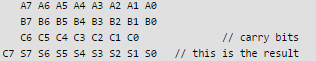So, it depends on S7 for overflow. when S7 is 0 while adding two negative numbers and when S7 is 1 while adding two positive numbers A7. B7. S7' + A7'. B7'.S7 = 1

QUESTION: 36

Consider a database that has the relation schemas EMP (EmpId, EmpName, DepId), and DEPT(DeptName, DeptId). Note that the DepId can be permitted to be NULL in the relation EMP. Consider the following queries on the database expressed in tuple relational calculus.

I. {t | ∃ u ∈ EMP (t[EMPName] = u[EmpName] ∧ ∀ v ∈ DEPT (t[DeptId] ≠ DeptId]))}
II. {t | ∃ u ∈ EMP (t[EMPName] = u[EmpName] ∧ ∃ v ∈ DEPT (t[DeptId] ≠ DeptId]))}
III. {t | ∃ u ∈ EMP (t[EMPName] = u[EmpName] ∧ ∃ v ∈ DEPT (t[DeptId] = DeptId]))}

Solution:

A SAFE EXPRESSION is one that is guaranteed to yield a finite number of tuples as its results. Otherwise, it is called UNSAFE Given, DepId can be permitted to be NULL

I. {t | ∃ u ∈ EMP (t[EMPName] = u[EmpName] ∧ ∀ v ∈ DEPT (t[DeptId] ≠ DeptId]))} : Gives empnames who donot belong to any department
II. {t | ∃ u ∈ EMP (t[EMPName] = u[EmpName] ∧ ∃ v ∈ DEPT (t[DeptId] ≠ DeptId]))} : Gives empnames who donot belong to some department
III. {t | ∃ u ∈ EMP (t[EMPName] = u[EmpName] ∧ ∃ v ∈ DEPT (t[DeptId] = DeptId]))}: Gives empnames who donot belong to same department
All of these queries are giving some results which are finite and thus all are safe expressions.
Therefore, option D is correct.

QUESTION: 37

Let G = (V, E) be any connected undirected edge-weighted graph. The weights of the edges in E are positive any distinct. Consider the following statements:

I. Minimum Spanning Tree of G is always unique.

II. Shortest path between any two vertices of G is always unique.

Q. Which of the above statements is/are necessarily true?

Solution:

I. Minimum Spanning Tree of G is always unique - MST will wlways be distinct if the edges are unique so Correct II. Shortest path between any two vertices of G is always unique - Shortest path between any two vertices can be same so incorrect Therefore, option A is correct

Alternate solution: We know that minimum spanning tree of a graph is always unique if all the weight are distinct, so statement 1 is correct. Now statement 2 , this might not be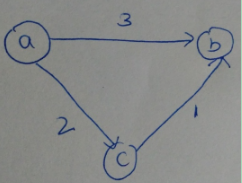true in all cases. Consider the graph.

There are two shortest paths from a to b (one is direct and other via node c) So statement 2 is false Hence option a is correct.

QUESTION: 38

Consider the expression (a-1) * ((( b + c ) / 3 )) + d)). Let X be the minimum number of registers required by an optimal code generation (without any register spill) algorithm for a load/store architecture, in which
(i) only load and store instructions can have memory operands and
(ii) arithmetic instructions can have only register or immediate operands
The value of X is ________.

Note: This questions appeared as Numerical Answer Type.

Solution:

The assembly code using the load/store architecture can be written as follows: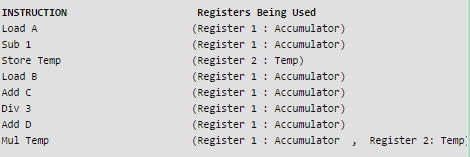QUESTION: 39

Consider the C functions foo and bar given below: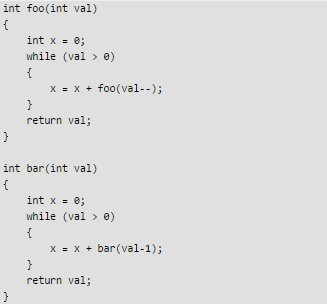Q. Invocations of foo(3) and bar(3) will result in:

Solution:

In the function foo every time in the while foo is called with the value 3 because val is passed with post decrement operator so the value 3 is passed and val is decremented later. Every time the function is called a new variable is created as the passed variable is passed by value, with the value 3. So the function will close abruptly without returning any value.
In the function bar, in the while loop value the value of val variable is not decrementing, it remains 3 only. Bar function in the while loop is called with val-1 i.e 2 but value of val is not decremented, so it will result in infinite loop.

QUESTION: 40

Let p, q, and r be the propositions and the expression (p -> q) -> r be a contradiction. Then, the expression (r -> p)-> q is

Solution:

The expression (r → p) → q is always true when q is true, regardless of the value of the expression (p → q) → r.

Alternate Solution

• Option (A): If (p → q) → r is false, then (p → q) is true and r is false.

The possible cases are
(i) p is true, q is true, r is false
(ii) p is false, q is true, r is false
(iii) p is false, q is false, r is false
For case (iii), (r → p) → q is false . It is not a tautology . Option (A) is not true.
• Option (B): For case (i) and case (ii), (r → p) → q is true.
It is not a contradiction , option (B) is not true.
• Option (C): For case (iii), p is false and (r → p) → q is also false
option (C) is not true.
• Option (D): Only for case (i) and case (ii), q is true For both cases, (r →p) →q is true. Option (D) is true
QUESTION: 41

Consider a RISC machine where each instruction is exactly 4 bytes long. Conditional and unconditional branch instructions use PC- relative addressing mode with Offset specified in bytes to the target location of the branch instruction. Further the Offset is always with respect to the address of the next instruction in the program sequence. Consider the following instruction sequence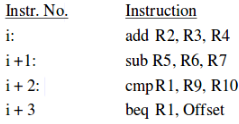Q. f the target of the branch instruction is i, then the decimal value of the Offest is __________.

Note: This questions appeared as Numerical Answer Type.

Solution: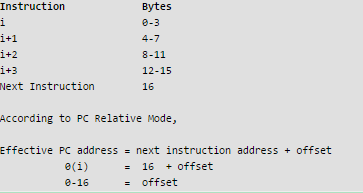QUESTION: 42

Consider the following grammar over the alphabet {a,b,c} given below, S and T are non-terminals.

G1: S-->aSb|T
T--> cT|∈

G2: S-->bSa|T
T--> cT|∈

Q.The language L1(G1) ∩ L2(G2).

Solution:

The language generated by grammar G1 is anc*bn where n>=0
The language generated by grammar G2 is bnc*an where n>=0
The intersection of two languages will be c* (putting n=0 in both languages)
We know that c* is a regular language and infinite, so option b is correct.

Alternate Solution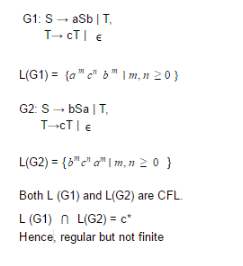QUESTION: 43

If G is grammar with productions

S → SaS | aSb | bSa | SS | ∈

Q. where S is the start variable, then which one of the following is not generated by G?

Solution: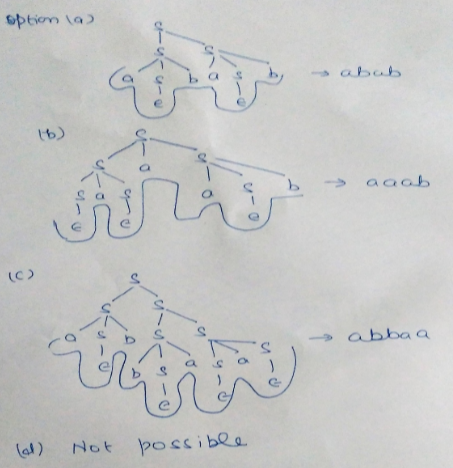QUESTION: 44

In a RSA cryptosystem a particular A uses two prime numbers p = 13 and q =17 to generate her public and private keys. If the public key of Ais 35. Then the private key of A is ____________.

Note: This questions appeared as Numerical Answer Type.

Solution:

n an RSA cryptosystem, for public key:
GCD( ϕ(n) , e) = 1

And, for private key:
(e * d) mod ϕ(n) = 1

Where,
ϕ(n) = (p -1)*(q - 1) = (13 - 1)(17 - 1) =12*16 = 192
Such that 1 < e, d < ϕ(n)

Therefore, the private key is:
(35 * d) mod ϕ(n) = 1
d = 11

QUESTION: 45

Let A be m×n real valued square symmetric matrix of rank 2 with expression given below.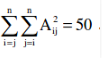Consider the following statements

(i) One eigenvalue must be in [-5, 5].
(ii) The eigenvalue with the largest magnitude must be strictly greater than 5.

Q. Which of the above statements about engenvalues of A is/are necessarily CORRECT?

Solution: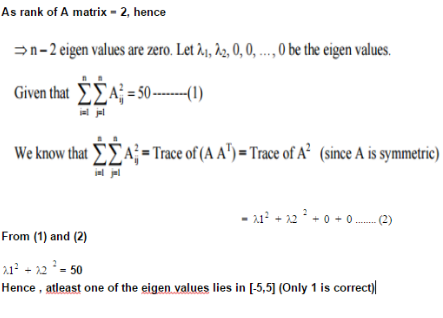QUESTION: 46

In a database system, unique time stamps are assigned to each transaction using Lamport’s logical clock. Let TS(T1) and TS(T2) be the time stamps of transactions T1 and T2 respectively. Besides, T1 holds a lock on the resource R, and T2 has requested a conflicting lock on the same resource R. The following algorithm is used to prevent deadlocks in the database assuming that a killed transaction is restarted with the same timestamp.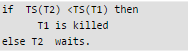Q. Assume any transactions that is not killed terminates eventually. Which of the following is TRUE about the database system that uses the above algorithm to prevent deadlocks?

Solution:

Given, if TS(T2) <TS(T1) then T1 is killed else T2 waits.

• T1 holds a lock on the resource R
• T2 has requested a conflicting lock on the same resource R

According to algo, TS(T2) <TS(T1) then T1 is killed else T2 will wait. So in both cases neither deadlock will happen nor starvation. Therefore, option A is correct

QUESTION: 47

The values of parameters for the Stop-and – Wait ARQ protocol are as given below.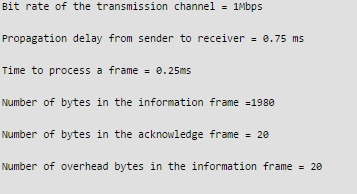Q. Assume that there are no transmission errors. Then the transmission efficiency (expressed in percentage) of the Stop-and – Wait ARQ protocol for the above parameters is _________( correct to 2 decimal place) .

Note: This questions appeared as Numerical Answer Type.

Solution:
QUESTION: 48

The value of the given expression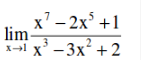Solution: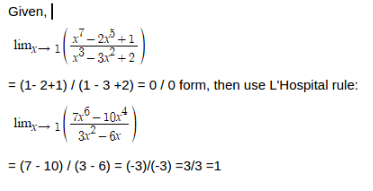QUESTION: 49

Consider the following two functions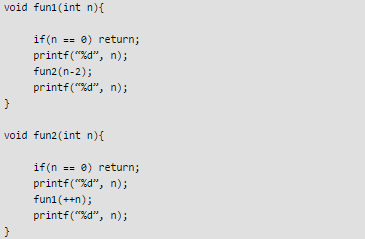Q. The output printed when fun1 (5) is called is

Solution: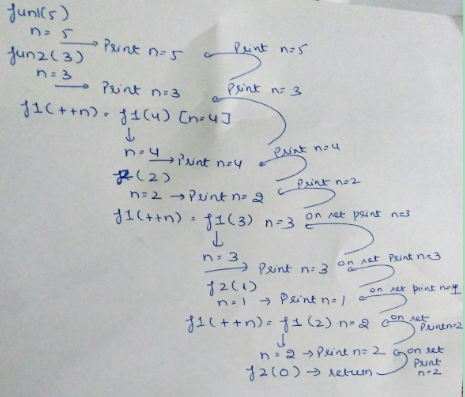Alternate Solution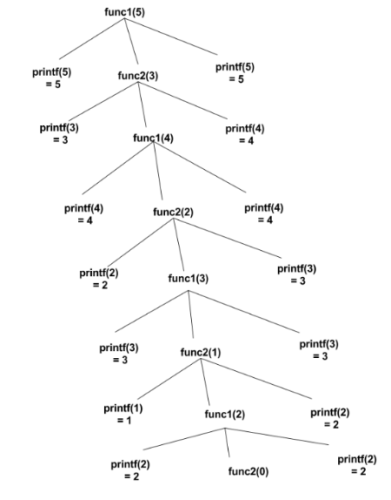QUESTION: 50

Consider a 2-way set associative cache with 256 blocks and uses LRU replacement, Initially the cache is empty. Conflict misses are those misses which occur due the contention of multiple blocks for the same cache set. Compulsory misses occur due to first time access to the block. The following sequence of accesses to memory blocks

(0,128,256,128,0,128,256,128,1,129,257,129,1,129,257,129)

is repeated 10 times. The number of conflict misses experienced by the cache is ___________.

Solution:
QUESTION: 51

A computer network uses polynomial over GF(2) for error checking with 8 bits as information bits and uses x3 + x + 1 as the generator polynomial to generate the check bits.
In this network, the message 01011011 is transmitted as

Solution:

We add (n-1) 0’s to the LSB of dividend(message) and divided by given GF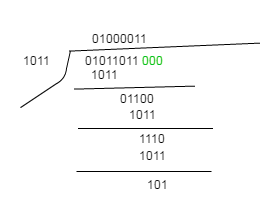The remainder will be CRC, it will be added to the LSB of the original message, that should be transmitted to the receiver. That is: 01011011101.

QUESTION: 52

Consider the following languages over the alphabet ∑= {a,b,c}. Let L1 = {an bncm | m, n >= 0 } and L2 = {ambncn| m, n >= 0}.

Q. Which of the following are context-free languages?
I. L1 ∪ L2
II. L1 ∩ L2

Solution:

Union of context free language is also context free language.
L1 = { an bn cm| m >= 0 and n >= 0 } and
L2 = { am bncn| n >= 0 and m >= 0 }
L3 = L1 ∪ L2 = { anbncm∪ ambncn| n >= 0, m >= 0 } is also context free.
L1 says number of a’s should be equal to number of b’s and L2 says number of b’s should be equal to number of c’s. Their union says either of two conditions to be true. So it is also context free language.
Intersection of CFG may or may not be CFG.
L3 = L1 ∩ L2 = { anbncn| n >= 0 } need not be context free.

QUESTION: 53

Consider the following grammar: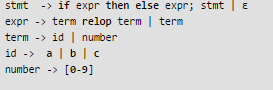where relop is a relational operate (e.g < >, ….), ε refers to the empty statement, and if ,thenelseare terminals. Consider a program P following the above grammar containing ten if terminals. The number of control flows paths in P is ____________. For example, the program

if e1 then e2 else e3

has 2 control flow paths, e1 -> e2 and e1 -> e3

Solution:
QUESTION: 54

A multithreaded program P executes with x number of threads and uses y number of locks for ensuring mutual exclusion while operating on shared memory locations. All locks in the program are non-reentrant, i.e., if a thread holds a lock l, then it cannot re-acquire lock l without releasing it. If a thread is unable to acquire a lock, it blocks until the lock becomes available. The minimum value of x and the minimum value of y together for which execution of P can result in a deadlock are:

Solution:

Reentrant (Recursive) locks allow a thread to reacquire the lock. That means same process can claim the lock multiple times without blocking on itself. This prevents the thread from deadlocking itself. This is main advantage of reentrant locks over non-reentrant locks.

Non-reentrant (Non- recursive) locks not allow a thread to reacquire the lock. That means same process can not claim the lock multiple times without releasing it. So, if a thread/process is unable to acquire a lock, it blocks until the lock becomes available. This situation is deadlocked.

Therefore, only one thread and only one lock can cause deadlock, if thread does try to reacquire lock.

When we have more than one process or one lock, then process/thread can acquire another lock to proceed further, or other process/thread can acquire lock to proceed further.

QUESTION: 55

Consider the following C program.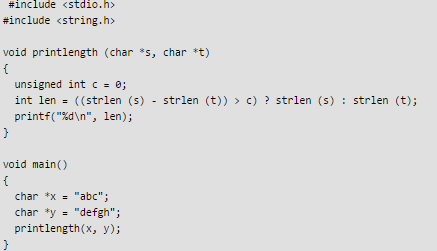Q. Recall that strlen is defined in string.h as returning a value of type size_t, which is an unsigned int . The output of the program is _________.

Note: This questions appeared as Numerical Answer Type.

Solution:

((strlen(s) - strlen(t)) > c) ? strlen (s) : strlen (t) = (3 - 5 > 0) = (-2 > 0) Important point here is while comparing -2 with c,  result will be a positive number as c is unsigned. So, out of these two , strlen (s) will be printed. Therefore, option B is correct. See the code for clarification :http://code.geeksforgeeks.org/hDPNVE

QUESTION: 56

A cache memory unit with capacity of N words and block size of B words is to be designed. If it is designed as direct mapped cache, the length of the TAG field is 10 bits. If the cache unit is now designed as a 16-way set-associative cache, the length of the TAG field is ______ bits.

Note: This questions appeared as Numerical Answer Type.

Solution:

Type of mapping is direct map; for this direct map, 10 bits are required in itss Tag.
It is updated to 16 way set Associative map then new tag field size = 10 + log216 = 14 bits, because for k way set associative map design, log2k bits are additionally required to the number of bits in tag field for Direct map design.

QUESTION: 57

Let A be an array of 31 numbers consisting of a sequence of 0’s followed by a sequence of 1’s. The problem is to find the smallest index i such that A[i] is 1 by probing the minimum number of locations in A. The worst case number of probes performed by an optimal algorithm is________.

Note: This questions appeared as Numerical Answer Type.

Solution:

The best way to solve such a problem is by using Binary Search. Search the sorted array by repeatedly dividing the search interval in half. Begin with an interval covering the whole array. If the value of the search key is less than the item in the middle of the interval, narrow the interval to the lower half. Otherwise narrow it to the upper half. Find mid element

• Is mid = 1 ?
• Is mid >1?(not possible here)
• Is mid < 1 ?

Proceed accordingly, Worst case of this problem will be 1 at the end of the array i.e 00000.....1 OR 1.......0000. It will take log n time worst case. n=31, Hence log 231 = 5. Therefore, option D is correct.

QUESTION: 58

The number of integers between 1 and 500 (both inclusive) that are divisible by 3 or 5 or 7 is ______.

Note: This questions appeared as Numerical Answer Type.

Solution:

The general formula for the union of 3 sets is:
(A union B union C) = A + B + C - (A intersect B) - (A intersect C) - (B intersect C) + (A intersect B intersect C).
Assuming,
A = 3, B = 5, C = 7
= 500/3 + 500/5 + 500/7 - 500/3*5 - 500/5*7 - 500/7*3 + 500/105
= 271
Therefore, option C is correct.
Alternate Solution: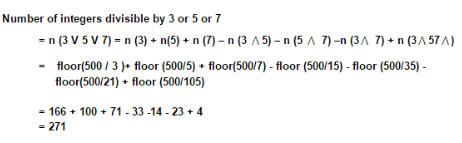Alternate Solution:

Alternate solution
Let a = number divisible by 3
b = number divisible by 5
c = number divisible by 7
n(a) = 166
n(b) = 100
n(c) = 71
n(a∩b) = number divisible by 15 = 33
n(b∩c) = number divisible by 35 = 14
n(a∩c) = number divisible by 21 = 23
n(a∩b∩c) = number divisible by 105 = 4
n(a∪b∪c) = n(a) + n(b) + n(c) - n(a∩b) - n(b∩c) - n(a∩c) + n(a∩b∩c) = 166 + 100 + 71 - 33 - 14 - 23 + 4 = 271

QUESTION: 59

Consider a database that has the relation schema CR(StudentName, CourseName). An instance of the schema CR is as given below.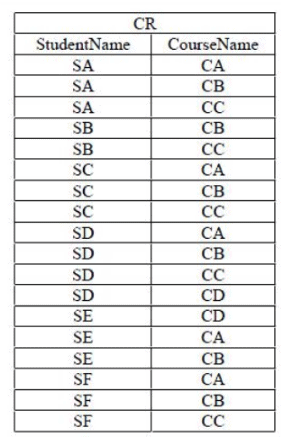Q. The following query is made on the database. T1 ← πCourseNameStudentName=’SA’(CR)) T2 ← CR ÷ T1 The number of rows in T2 is ________.

Note: This questions appeared as Numerical Answer Type.

Solution:

Result of T1: CA CB CC Result of T2 = CR/T1 SA SC SD SF Total = 4 Therefore, option C is correct

Alternate Solution :
The output of query T1 will be the courses enrolled by student SA i.e CA , CB, CC
The output of query T2 will be the names of the students who enrol all the courses that are the output of query T1 (division operator) i.e CA, CB, CC
From the table we can see that SA, SC, SD, SF have enrolled all the courses so the output of T2 will have 4 rows.

QUESTION: 60

Consider a combination of T and D flip-flops connected as shown below. The output of the D flipflop is connected to the input of the T flip-flop and the output of the T flip-flop is connected to the input of the D flip-flop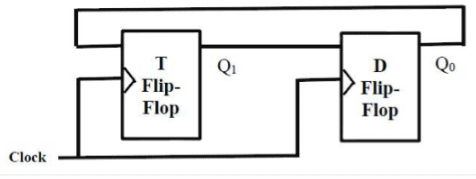Q. Initially, both Q0 and Q1 are set to 1 (before the 1st clock cycle). The outputs

Solution: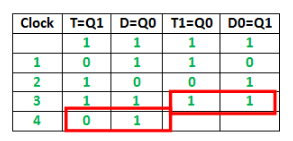It can be observed from the diagram that : Q1 Q0 after the 3rd cycle are 11 and after the 4th cycle are 01 Therefore, option B is correct

QUESTION: 61

Let u and v be two vectors in R2 whose Euclidean norms satisfy |u| = 2|v|. What is the value α such that w = u + αv bisects the angle between u and v ?

Solution:

|u| = 2|v| =|2v|. So u and 2v are vectors of the same length in directions of u and v, so their sum u+2v bisects the angle.
w = u + αv = u + 2 v
That is α = 2
Because, If we have two vectors with equal magnitude in the direction of given vectors, then their sum will bisect the angle between them.

QUESTION: 62

Let A and B be infinite alphabets and let # be a symbol outside both A and B. Let f be a total functional from A* to B* .We say f is computable if there exists a Turning machine M which given an input x in A*, always halts with f(x) on its tape. Let Lf denotes the language {x#f(x)|x∈A*}. Which of the following statements is true?

Solution:

This definition is given as Halting of Turing Machine. Every recursive language is computable, but converse may not be true.

QUESTION: 63

Recall that Belady’s anomaly is that the pages-fault rate may increase as the number of allocated frames increases. Now consider the following statements: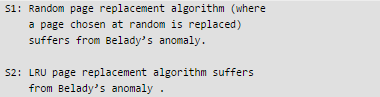Q. Which of the following is CORRECT?

Solution:

Belady’s anomaly proves that it is possible to have more page faults when increasing the number of page frames while using the First in First Out (FIFO) page replacement algorithm. For example, if we consider reference string 3 2 1 0 3 2 4 3 2 1 0 4 and 3 slots, we get 9 total page faults, but if we increase slots to 4, we get 10 page faults.

S1: Random page replacement algorithm (where a page chosen at random is replaced) suffers from Belady’s anomaly. -> Random page replacement algorithm can be any including FIFO so its true

S2: LRU page replacement algorithm suffers from Belady’s anomaly . -> LRU does'nt suffer from Belady’s anomaly .

Therefore, option B is correct

QUESTION: 64

The output of executing the following C program is ________.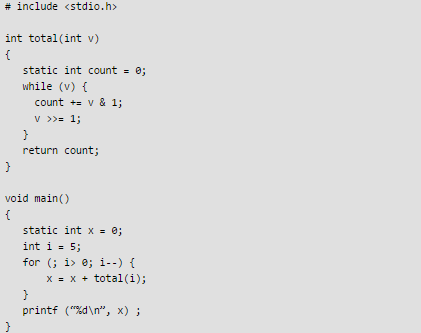Solution:

Digits : 5-0101, 4-0100, 3-0011, 2-0010, 1-0000 Countof 1s : 2, 3, 5, 6, 7 Total: 2+3+5+6+7 = 23 total(i)=23 Therfore, option A is correct Check out the running code with comments: [sourcecode language="CPP"] #include int total(int v) { static int count = 0; while(v) { count += v&1; v >>= 1; } //This count can be used to see number of 1s returned //for every number i //printf("%d", count); return count; } void main() { static int x=0; int i=5; for(; i>0; i--) { //total gets added everytime with total number //of digits in the given number i x = x + total(i); } printf("%d ", x); } [/sourcecode]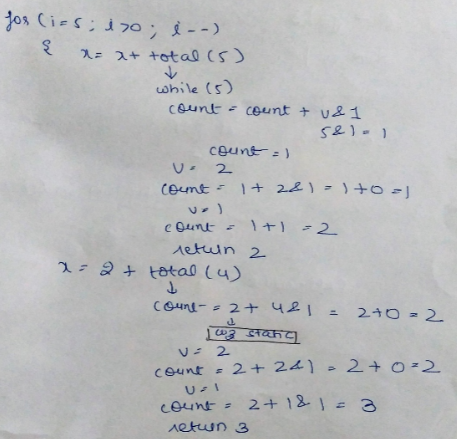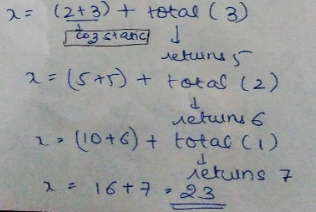QUESTION: 65

Instruction execution in a processor is divided into 5 stages. Instruction Fetch(IF), Instruction Decode (ID), Operand Fetch(OF), Execute(EX), and Write Back(WB), These stages take 5,4,20, 10 and 3 nanoseconds (ns) respectively. A pipelined implementation of the processor requires buffering between each pair of consecutive stages with a delay of 2 ns. Two pipelined implementations of the processor are contemplated:
(i) a naïve pipeline implementation (NP) with 5 stages and
(ii) an efficient pipeline (EP) where the OF stage id divided into stages OF1 and OF2 with execution times of 12 ns and 8 ns respectively.

The speedup (correct to two decimals places) achieved by EP over NP in executing 20 independent instructions with no hazards is ________________.

Note: This questions appeared as Numerical Answer Type.

Solution:

Given, total number of instructions (n) = 20
For naive pipeline (NP):

Number of stages(k) = 5 Clock time (Tp) = max {(stage delay+buffer delay)} = {7, 6, 22, 12, 5} = 22 nsec
Execution time (Enp) = (k + n - 1)*Tp = (5 + 20 - 1)*22 = 528 nsec

For efficient pipeline (EP):

number of stages(k) = 6 (delay with 20 nsec stage is divided into 12 nsec and 8 nsec)
Clock time (Tp) = max {(stage delay+buffer delay)} = {7, 6, 14, 10, 14, 5} = 14 nsec
Execution time (Eep) = (k + n - 1)*Tp = (6 + 20 - 1)*14 = 350 nsec

Therefore, Speedup = (Enp) / (Eep) = 528 / 350 = 1.508